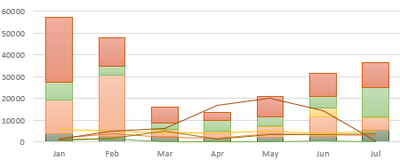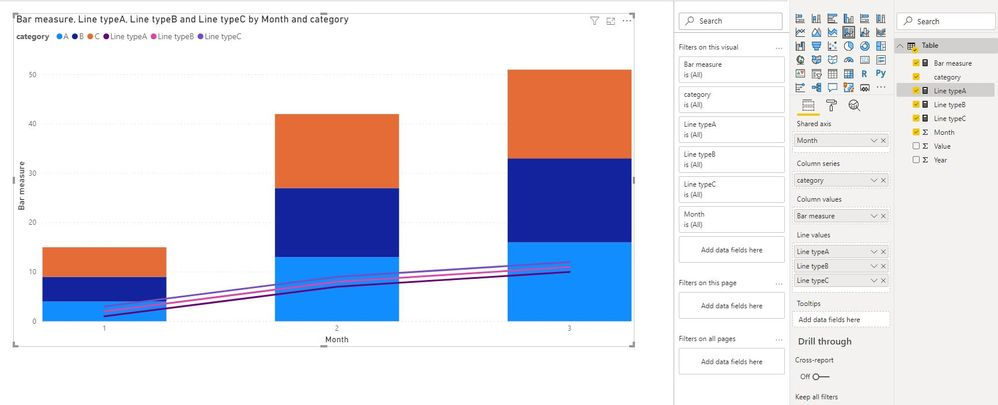cancel
Showing results for
Did you mean:Helper V

## Looking for equivalent of Line-Bar combo in Excel to PowerBI

Hello!

I'm new to PowerBI (currently exploring) and I'm looking for possible replacement to the chart below:It is made in excel where the line chart pertains to the 2019 data and the bars for the 2020 data. There are only 3 data columns used: a measure, a category (food type, corresponds to the colors of both the line and bars) and a date data.

I believe this is a bad way of representing data because it is quite confusing to the readers so I'm looking for another way to interpret it. There is no clustered stacked bars nor line-bar chart on PowerBI so my question is, is there another visual for this requirement? (I also tried hierarchy - I made a type & date hierarchy and placed it on the x-axis but it isn't right).

1 ACCEPTED SOLUTIONCommunity Support

To my knowledge, there is no visuals like this, but there is a workaround to get it.

Step1:

Create a measure for column

Bar measure = CALCULATE(SUM('Table'[Value]),FILTER('Table','Table'[Year]=2020))
Step2:
Create measure for different category

Line typeA = CALCULATE(SUM('Table'[Value]),FILTER('Table','Table'[Year]=2019&&'Table'[category ]="A"))

Line typeB = CALCULATE(SUM('Table'[Value]),FILTER('Table','Table'[Year]=2019&&'Table'[category ]="B"))

Line typeC = CALCULATE(SUM('Table'[Value]),FILTER('Table','Table'[Year]=2019&&'Table'[category ]="C"))

Step3:

Now create a visual like thisand here is sample pbix file, please try it.

Regards,

Lin

Community Support Team _ Lin
If this post helps, then please consider Accept it as the solution to help the other members find it more quickly.
4 REPLIES 4Community Support

To my knowledge, there is no visuals like this, but there is a workaround to get it.

Step1:

Create a measure for column

Bar measure = CALCULATE(SUM('Table'[Value]),FILTER('Table','Table'[Year]=2020))
Step2:
Create measure for different category

Line typeA = CALCULATE(SUM('Table'[Value]),FILTER('Table','Table'[Year]=2019&&'Table'[category ]="A"))

Line typeB = CALCULATE(SUM('Table'[Value]),FILTER('Table','Table'[Year]=2019&&'Table'[category ]="B"))

Line typeC = CALCULATE(SUM('Table'[Value]),FILTER('Table','Table'[Year]=2019&&'Table'[category ]="C"))

Step3:

Now create a visual like thisand here is sample pbix file, please try it.

Regards,

Lin

Community Support Team _ Lin
If this post helps, then please consider Accept it as the solution to help the other members find it more quickly.Helper V

HI @v-lili6-msft , thanks for the reply. I applied your solution and it solved my problem (although I admit there's a more better way to represent my data instead of line-chart combo). Will close this thread now.Super UserHelper V

Hi @amitchandak ! I also tried this but I still need my line chart to be a multiple line chart because I need to show the composition. Still, thanks for link!Announcements#### Exclusive opportunity for Women!

Join us for a free, hands-on Microsoft workshop led by women trainers for women where you will learn how to build a Dashboard in a Day!#### Power Platform Conference-Power BI and Fabric Sessions

Join us Oct 1 - 6 in Las Vegas for the Microsoft Power Platform Conference.Top Solution Authors
Top Kudoed Authors
Users online (2,592)